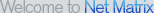# Free Math Downloads## Download Math Software

More Math Software: 23
Page / 1 / 2 / 3

Algebra - One On One
Algebra - One on One is an educational game for those wanting a fun way to learn and practice algebra. This program covers 21 functions. It has a practice and a game area. Includes a two player combat game.

Arcs & Sectors
Middle-School (grades 5 through 9) math program written to provide skills in context. Given the radius or diameter of a circle, and the measure of a central angle, students find various areas and lenths.

Box & Whiskers Drill
Middle-School (grades 5 through 9) math program written to provide skills in context. Students use sets of data to construct on-screen box and whisker plots.

Calc 3D
Calc3D is a calculator for vectors, matrices, complex numbers, quaternions and coordinates. For objects like point, line, plane and sphere distances, intersections, volume, area of squeres, area of a triangle can be calculated. (5 languages)

Calc 3D Pro
Calculator for vectors, matrices, complex numbers, coordinates, intersections and function plotting. For objects like point, line, plane and sphere distances, intersections, volume, area of squeres, area of a triangle can be calculated.

Flashcard Tables
Select which of the tables you want to practice, and the program will present them as flashcards. See how quickly you can answer and get listed in the hall of fame. Demo limited to 1s, 2s and 3s table. Statistics saved about each run.

Functions
Visualizes and studies functions of one variable to find roots, extrema, integral, derivatives, graph. Results can be saved or printed. You can copy the graph to the clipboard. You have one-click control of the graph with zooming, panning, centering

GEUP
GEUP is an interactive, powerful and easy to use software for learning and doing Geometry on the computer. It allows to verify geometric properties in a precise way and to discover new properties through the exploration.

License Plate Math
License Plate Math is a fun game you can play on the road as well as on the computer. There is a virtually unlimited number of math puzzles, with 3 ways to play. The 11 levels of difficulty ensure continuing challenge. For ages 9 and up.

Linear Algebra
Performs computations associated with matrices: Solves systems of linear equations, finds sum, difference, product, determinant, trace, inverse, adjoint, transpose, eigenvalues, eigenvectors, LU factors, QR factors, tests for definiteness, etc.

More Math Software: 23
Page / 1 / 2 / 3